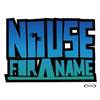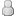• 260 Vote(s) - 2.79 Average
• 1
• 2
• 3
• 4
• 508-29-2011, 09:51 AM,thiguchiJunior MemberReputation: 2 Posts: 8 Joined: Jul 2011
ŃüōŃéōŃü½ŃüĪŃü»ŃĆéĶ│¬ÕĢÅŃü¦ŃüÖ’╝ü

ŃéłŃéŹŃüŚŃüÅŃüŖķĪśŃüäŃüŚŃüŠŃüÖ’╝ü

Code:
```{import * from CURL.GUI.CHARTS} {let records:RecordSet =     {RecordSet         {RecordFields             {RecordField "KPH", caption = "Kilometers/hour", domain = int},             {RecordField "TD", caption = "Thinking distance", domain = int},             {RecordField "BD", caption = "Braking distance", domain = int},             {RecordField "OD", caption = "Overall distance", domain = int}         },         {RecordData KPH = 32, TD = 6, BD = 6, OD = 12},         {RecordData KPH = 48, TD = 9, BD = 14, OD = 23},         {RecordData KPH = 64, TD = 12, BD = 24, OD = 36},         {RecordData KPH = 80, TD = 15, BD = 38, OD = 53},         {RecordData KPH = 97, TD = 18, BD = 55, OD = 73},         {RecordData KPH = 113, TD = 21, BD = 75, OD = 96}     } } {let chart:LayeredChart =     {LayeredChart         width = 15cm,         height = 6cm,         plot-area = {LayeredPlotArea                         right-axis-grid-lines? = true                     },         left-axis =             {ChartAxis                 {ChartDataSeries records, "OD"},                 axis-label = "OD"             },         right-axis =             {ChartAxis                 {ChartDataSeries records, "BD"},                 axis-label = "BD"             },         bottom-axis =             {ChartAxis                 {ChartDataSeries records, "KPH"},                 force-zero? = false             },         {LineLayer             {ChartDataSeries records, "OD"},             {ChartDataSeries records, "BD"},             {ChartDataSeries records, "TD"}         }     } } {VBox     {RecordGrid         height = 4cm,         width = 13cm,         record-source = records     },     chart }```
08-29-2011, 02:11 PM,c-sCurl EngineerReputation: 11 Posts: 57 Joined: Jul 2011
ŃüōŃüåŃüÖŃéīŃü░Õ”éõĮĢŃü¦ŃüŚŃéćŃüåŃüŗŃĆé

Code:
```{curl 7.0, 8.0 applet} {import * from CURL.GUI.CHARTS} {define-class public open MyLayeredPlotArea {inherits LayeredPlotArea}      {constructor public {default                           grid-line-group:Shape = {ShapeGroup},                           left-grid-line-group:Shape = {ShapeGroup},                           right-grid-line-group:Shape = {ShapeGroup},                           top-grid-line-group:Shape = {ShapeGroup},                           bottom-grid-line-group:Shape = {ShapeGroup},                           color:FillPattern = FillPattern.silver,                           ...                       }     {construct-super         grid-line-group = grid-line-group,         left-grid-line-group = left-grid-line-group,         right-grid-line-group = right-grid-line-group,         top-grid-line-group = top-grid-line-group,         bottom-grid-line-group = bottom-grid-line-group,         color = color,         ...     }   }      {method protected open {generate-grid-lines                              container:Shape,                              axis:ChartAxis,                              horizontal?:bool                          }:void     {if axis.position == AxisPosition.right then         {for tick in {axis.get-major-ticks-Iterator} do             let constant pos:Distance = tick.position             let p1:Distance2d = {Distance2d 0m, 0m}             let p2:Distance2d = {Distance2d 0m, 0m}             set p1 = {Distance2d -self.width, pos}             set p2 = {Distance2d 0m, pos}             set p1 = {axis.transform-to-chart p1}             set p2 = {axis.transform-to-chart p2}             set p1 = {self.transformation.inverse-transform-point p1}             set p2 = {self.transformation.inverse-transform-point p2}                          {container.add {PathShape {Path p1, PathOperation.line-to, p2}}}         }     else         {super.generate-grid-lines             container,             axis,             horizontal?         }     }   }    } {let records:RecordSet =     {RecordSet         {RecordFields             {RecordField "KPH", caption = "Kilometers/hour",   domain = int},             {RecordField "TD",  caption = "Thinking distance", domain = int},             {RecordField "BD",  caption = "Braking distance",  domain = int},             {RecordField "OD",  caption = "Overall distance",  domain = int}         },         {RecordData KPH =  32, TD =  6, BD =  6, OD = 12},         {RecordData KPH =  48, TD =  9, BD = 14, OD = 23},         {RecordData KPH =  64, TD = 12, BD = 24, OD = 36},         {RecordData KPH =  80, TD = 15, BD = 38, OD = 53},         {RecordData KPH =  97, TD = 18, BD = 55, OD = 73},         {RecordData KPH = 113, TD = 21, BD = 75, OD = 96}     } } {LayeredChart     width = 15cm,     height = 6cm,     plot-area =         {MyLayeredPlotArea             left-axis-grid-lines? = false,             right-axis-grid-lines? = true         },     left-axis =         {ChartAxis             {ChartDataSeries records, "OD"},             axis-label = "OD"         },     right-axis =         {ChartAxis             {ChartDataSeries records, "BD"},             axis-label = "BD"         },     bottom-axis =         {ChartAxis             {ChartDataSeries records, "KPH"},             force-zero? = false         },     {LineLayer         {ChartDataSeries records, "OD"},         {ChartDataSeries records, "BD"},         {ChartDataSeries records, "TD"}     } }```
 « Next Oldest | Next Newest »

Forum Jump: